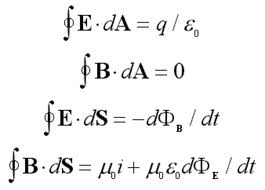# to model a "displacement current force" (Maxwell)

The Biot-Savart law describes the magnetic field force (Maxwell), calculated from a electric current density (or the flow/current field (Navier), calculated from a vortex field). The current density (not the origin current force) is "generated" by:

- a "truly" electric current

- a mathematically required "change" of the electric field.

The "route cause" of electric current in the Biot-Savart law is captured by the magnetic constant.

The later one is a (purely mathematically required) arte-fact, which got the (a posteriori) physical interpretation of a Maxwell (displacement) current; this is similar to the a posteriori "defined" (boundary layer created) vortex in an a priori vortex-free "ideal" fluid framework with related (vortex-free) potential streams). The mathematical tool is mainly about the Stokes theorem, which anticipates the "standard" normal derivative and its related regularity conditions.

The Stokes theorem in its integral form is most directly linked to the comments of the tab "J. Plemelj and ..." with respect to a a more general concept of a "mass element" and a "potential". Following those conceptual ideas/proposals, linking it with the proposed new energy inner product/ norm, it leads to a varaitonal representation of the Maxwell equations in a "less regular" (distributional) Hilbert space framework with corresponding less regular energy Hilbert space than H(1).